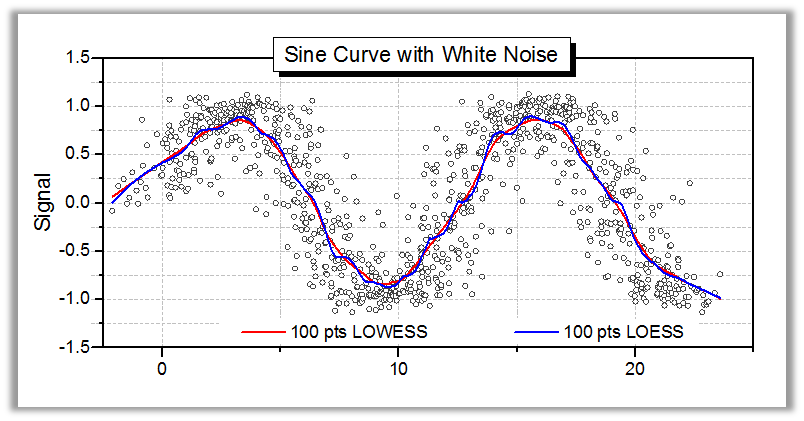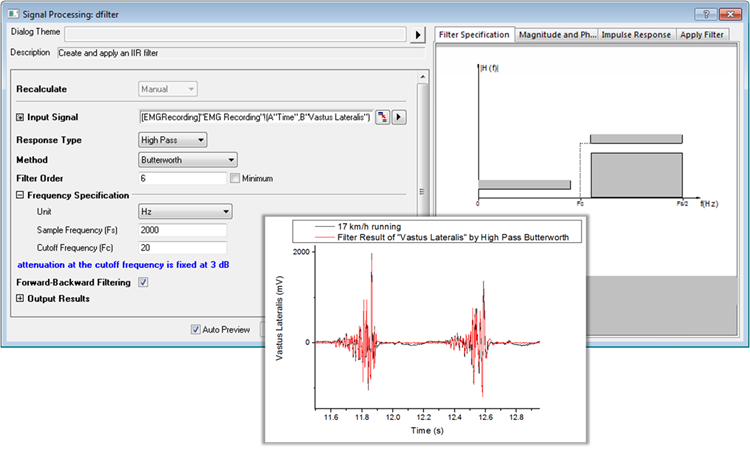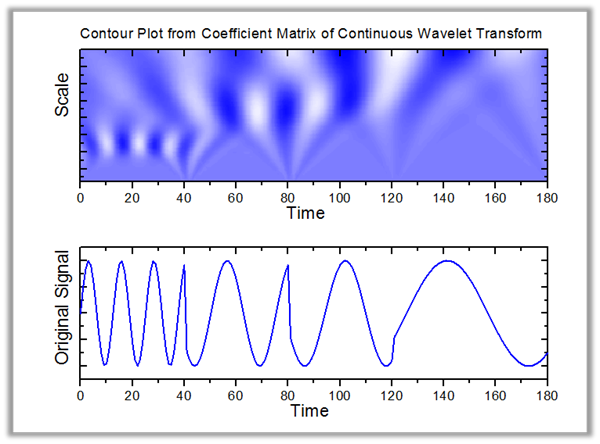# Signal Processing

• You are here:
• Home
• /
• PRODUCTS
• /
• Origin
• /
• Signal Processing

Origin은 다양한 신호 처리 도구를 제공합니다.

### Transforms

• Fast Fourier Transform and        Inverse Fast Fourier Transform (FFT / IFFT)
• Short-time Fourier Transform (STFT) PRO
• 2D FFT / 2D IFFT PRO
• Hilbert Transform PRO

Short-Time Fourier Transform (STFT) tool 은 비정상적인 신호에 대한 시간 · 주파수 분석을 실시합니다.

Continuous Wavelet Transform 을 이용한 시간 주파수 앱 (Time-Frequency Analysis App) 도 사용할 수 있습니다.

### Smmothing

• Savitzky-Golay
• Adjacent-Averaging
• FFT Filter
• Percentile Filter
• Lowess and Loess◀ Origin 의 Smooth tool 은 노이즈 제거를 위한 Adjacent Averaging, Savitzky-Golay, Percentile Filter, FFT Filter, LOWESS, LOESS, Binomail Method 등의 스무딩 기법 을 제공합니다.

이 그래프는 노이즈 데이터의 경향을 찾는 데 유효한 LOWESS 와 LOESS 스무딩 결과를 보여줍니다.

### Filtering

• FFT Filter
• IIR Filter Design PRO
• 2D FFT Filter PRO◀ OriginPro 는 무한 임펄스 응답 (Infinite Impulse Response <IIR>) 필터 를 제공하고, IIR 디지털 필터의 설계, 분석을 할 수 있습니다.

필터링 방법은 Butterworth, Chebyshev 유형 I 및 II, 타원 (Eliptic) 등의 방법이 준비되어 있습니다.  미리보기 패널에서는 지정된 매개 변수와 그 결과를 실시간으로 시각화하여 볼 수 있습니다.

### Wavelet Analysis

• Continuous Wavelet Transform PRO
• Discrete Wavelet Transform (Decomposition) PRO
• Inverse Descrete Wavelet Transform (Reconstruction) PRO
• Multi-Scale Wavelet Decomposition PRO
• Smoothing PRO
• Noise Removal PRO
• 2D Wavelet Decomposition PRO
• 2D Wavelet Reconstruction PRO◀ OriginPro 는 Wavelet Transform tools 를 제공합니다.

이 그래프는 Continuous Wavelet Transform (CWT) 도구를 사용하여 얻은 1D signal wavelet coefficients 를 보여줍니다.  또한 Time-Frequency Analysis App 도 제공되며, OriginLab의 File Exchange 사이트에서 다운로드 할 수 있습니다.

### Additional Tools

• Convolution
• Correlation
• Coherence PRO
• Envelope Curves PRO
• Decimation PRO
• Rise Time Gadget PRO